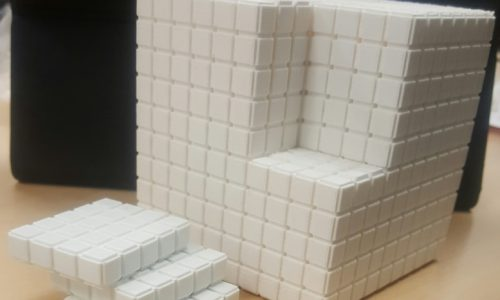# “The Hexahedron Cube”The manipulative we are going to make is a 10x10x10 cube that will help students learn multiplication by counting the cubes. A cube has length, width, and height, and with our cube manipulative children will be able to learn how to multiply two numbers by length x width by counting the number of cubes in the rows and columns. This will also introduce them to the area of a cube. This will also apply to three numbers that will include length x width x height that will teach them the volume of a cube. In all the children, will learn the multiplication of 2 and 3 numbers together. As the children count the blocks, they will be learning as well as gearing their interest into the cube and wanting to become engaged in how to multiply.

How the user of your manipulative is supposed to learn from working with your design?

The purpose of the cube is to teach children how to multiply 2 and 3 numbers using this cube. The 10x10x10 cube is designed such that the entire cube will have sub cubes of 1x1x1. The child will then count the number of small blocks/cubes to multiply.

How does the design of your technology reflect your understanding of what mathematics is and of how learning happens?

Our design reflects learning using direct interaction in the environment and using our cube. We have created an abstract structure that has attributes and properties that will help children learn to multiply, and helps them in the cognitive process of learning.  Our technology design will keep children engaged, while also increasing their interest and enjoyment in understanding math. Learning happens when children begin to have conceptual understanding. When we allow for the student to work with our cube we want them to develop ideas, and create their own strategies for learning and understanding the concept we present to them. Learning and understanding math also happens when there is confidence. Our main priority for students using the manipulatives is to feel confident that they will be able to multiply, and find this learning fun and resourceful.

What would it mean for your project to be successful in terms of learning?

Our projects success is based on how well the children respond to the manipulative design. In terms of learning the students would need to reflect that they understand what is presented before them, and that our cubes engage them. In an effort for the students to learn our cube needs to have the proper content that will allow the children to be able to learn to multiply. The tool will need to be meaningful to their learning and understanding of how to multiply two and three numbers. This projects success is also based on how the students can make connections from the multiplications to other areas, as well as the strategies they could come up with. If the project is successful, the students will build multiplication skills. They should also build inquiry from it. The students should be able to reflect on what they have gathered from working with the manipulative, how they have learned from it, and how they can use this to help them with everyday multiplication academics.  The success of the cube would finally come from how the student explanation of how they learned. If the manipulative was well understood, we will also check for understanding when the tool is not being used.

What would it mean if it were to fail to work?

If the tool failed to work it would mean that maybe the cube was confusing for the student to understand. It could also mean that it did not meet the expectations we were hoping for, and that we did not foresee future setbacks with the cube. It could also mean that the cube was not at the child’s developmental level. Another possible reason it failed to work would be that it could have been time consuming.

What kind of testing could you imagine carrying out for your design?

We are planning to do below two test cases:

1. Verify multiplication of 10×10 holds. We will pick any one face of the 10x10x10 cube and count the total number of 1x1x1 sub cubes in it. There should be a total of 100 cubes.
2. Verify multiplication of 3x4x5 holds. We will carve out a 3x4x5 cross section and then further divide it into three 1x4x5 stackable cubes. Upon physical counting, there should be a total of sixty 1x1x1 cubes, which would verify that multiplication holds.